# 竞赛：糖尿病遗传风险检测挑战赛（科大讯飞）

• 2022 年 8 月 02 日
• 本文字数：5441 字

阅读完需：约 18 分钟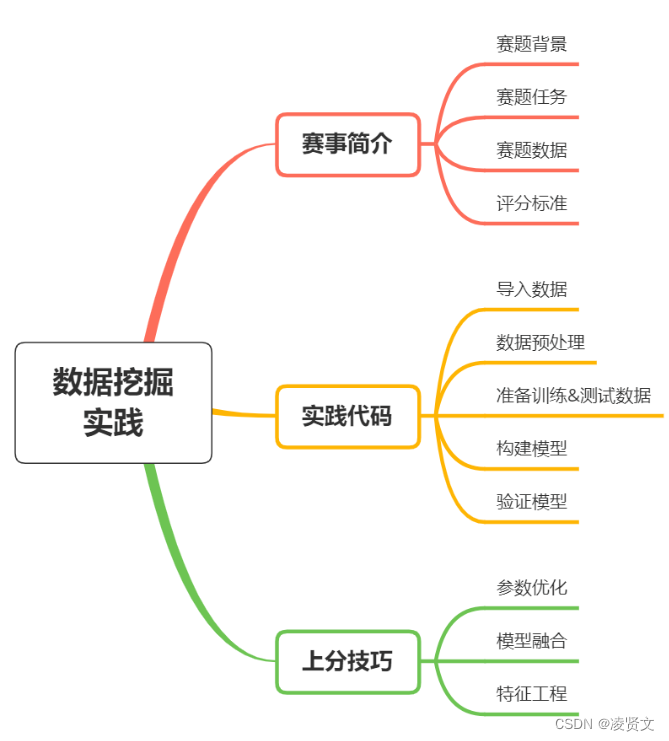uuid,label

1,0

2,1

3,1

...

# 四、评估指标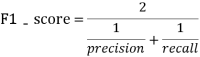​其中：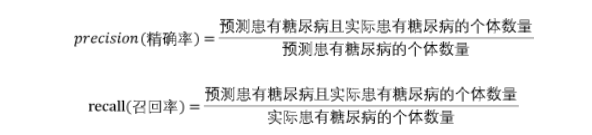# 五、数据分析

## 5.1 导入数据

import pandas as pdtrain_df = pd.read_csv('./糖尿病遗传风险预测挑战赛公开数据/比赛训练集.csv', encoding='gbk')test_df = pd.read_csv('./糖尿病遗传风险预测挑战赛公开数据/比赛测试集.csv', encoding='gbk')print(train_df.shape, test_df.shape)print(train_df.dtypes, test_df.dtypes)

## 5.2 查看训练集和测试集字段类型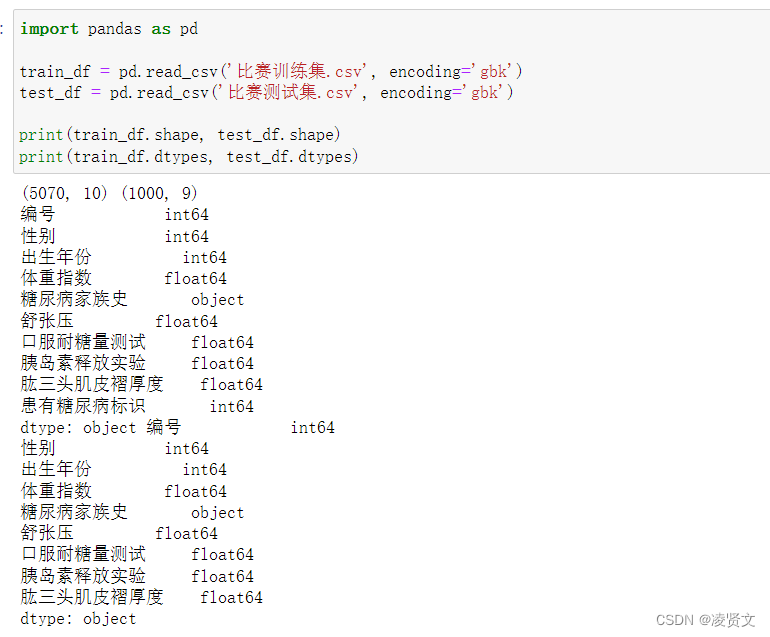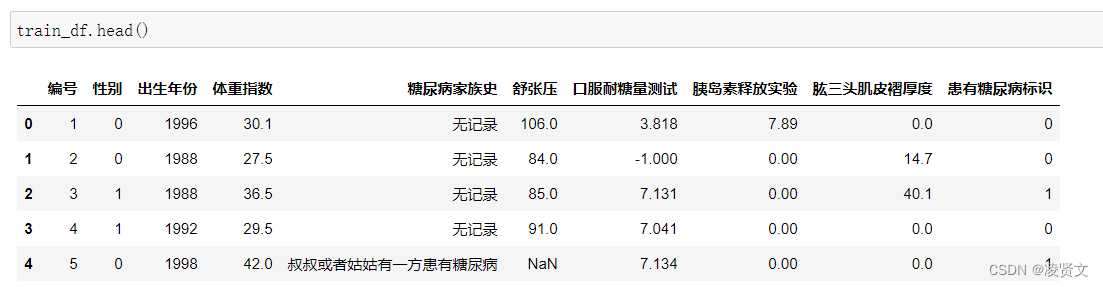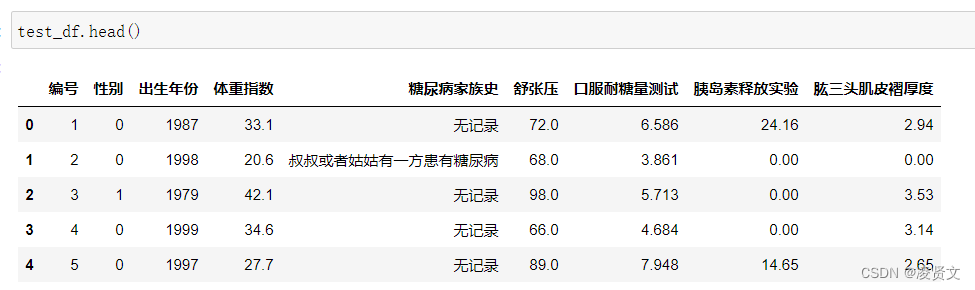## 5.3 统计字段的缺失值

train_df.isnull().sum()

编号            0性别            0出生年份          0体重指数          0糖尿病家族史        0舒张压         247口服耐糖量测试       0胰岛素释放实验       0肱三头肌皮褶厚度      0患有糖尿病标识       0dtype: int64

test_df.isnull().sum()

编号           0性别           0出生年份         0体重指数         0糖尿病家族史       0舒张压         49口服耐糖量测试      0胰岛素释放实验      0肱三头肌皮褶厚度     0dtype: int64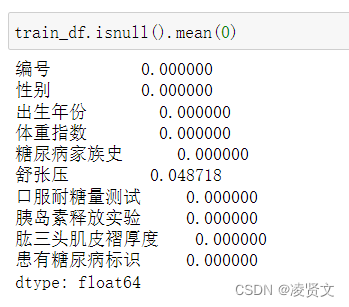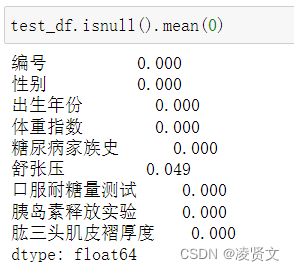## 5.4 分析字段类型。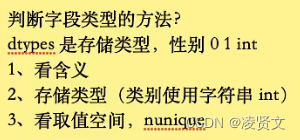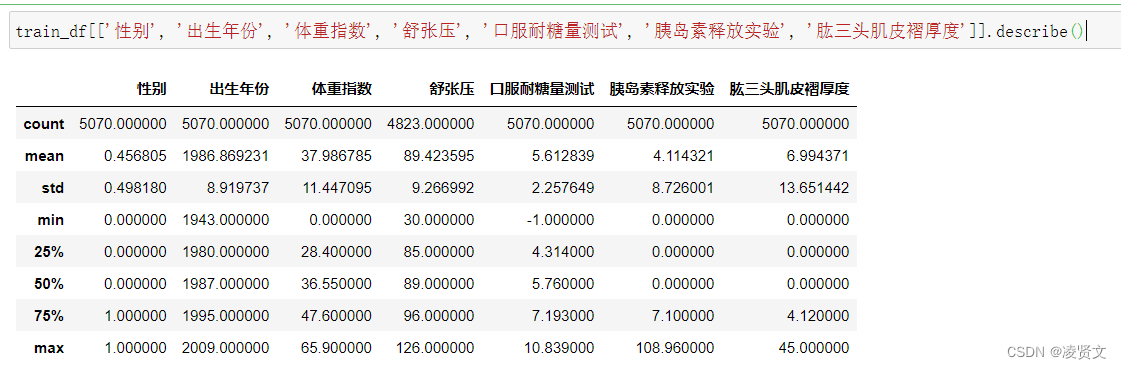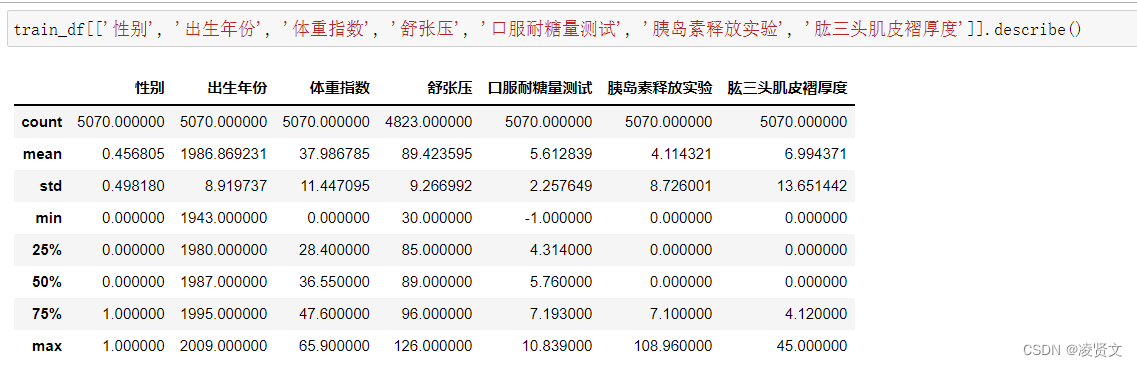## 5.5 字段相关性

import matplotlib.pyplot as pltimport seaborn as snsplt.rcParams['font.sans-serif'] = ['FangSong']  # 用来正常显示中文标签plt.rcParams['axes.unicode_minus'] = False  # 用来正常显示负号# 训练集相关性热力图矩阵plt.subplots(figsize=(10,10))sns.heatmap(train_df.corr(method='pearson'), annot=True, vmax=1, square=True, cmap='YlGnBu')plt.savefig('train_pearson.jpg', dpi=800)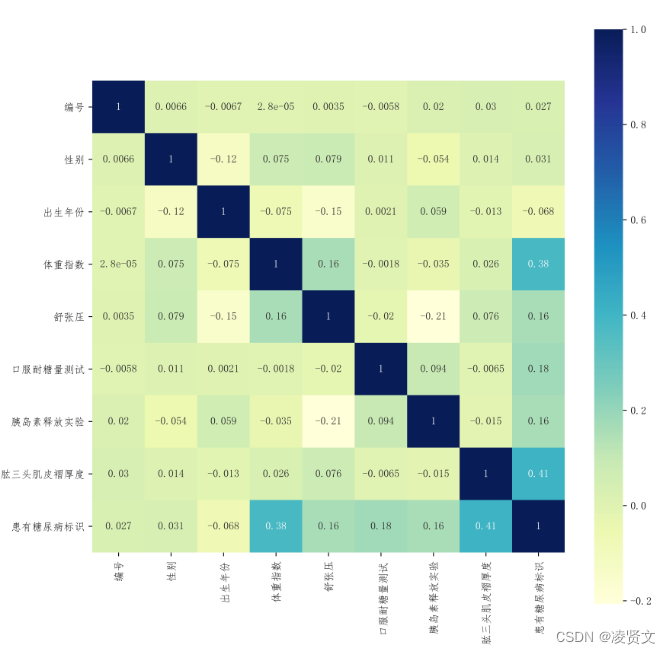# 测试集相关性热力图矩阵plt.subplots(figsize=(10,10))sns.heatmap(test_df.corr(method='pearson'), annot=True, vmax=1, square=True, cmap='YlGnBu')plt.savefig('test_pearson.jpg', dpi=800)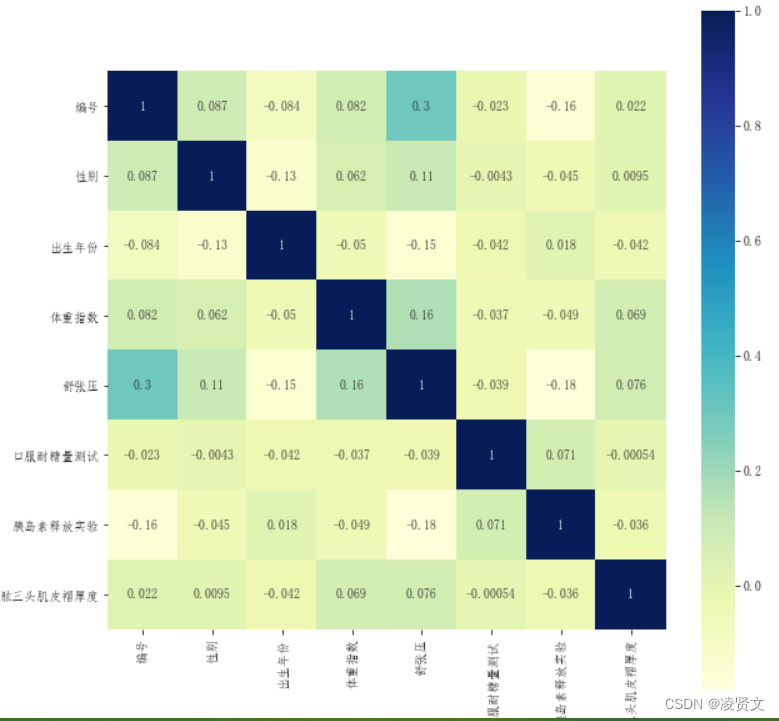# 六、逻辑回归尝试

## 6.1 导入 sklearn 的逻辑回归

# 构建逻辑回归模型import numpy as npfrom sklearn.linear_model import LogisticRegressionfrom sklearn.tree import DecisionTreeClassifierfrom sklearn.preprocessing import MinMaxScalerfrom sklearn.pipeline import make_pipeline

# 构建逻辑回归模型model = make_pipeline(    MinMaxScaler(),    LogisticRegression())model.fit(train_dataset,train_data["患有糖尿病标识"])

## 6.2 使用训练集和逻辑回归进行训练，并在测试集上进行预测

test_dataset["label"] = model.predict(test_dataset.drop(["编号"],axis=1))test_dataset.rename({"编号":'uuid'},axis=1)[['uuid','label']].to_csv("submit_lr.csv",index=None)

## 6.3 提交结果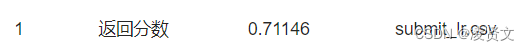## 6.4 尝试决策树模型

# 尝试构建决策树模型model = make_pipeline(    MinMaxScaler(),    DecisionTreeClassifier()    )model.fit(train_dataset,train_data["患有糖尿病标识"])test_dataset["label"] = model.predict(test_dataset.drop(["编号",'label'],axis=1))test_dataset.rename({"编号":'uuid'},axis=1)[['uuid','label']].to_csv("submit_dt.csv",index=None)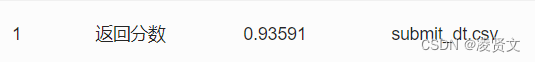# 七、特征工程

## 7.1 统计每个性别对应的[体重指数]、[舒张压]平均值

train_dataset.groupby("性别")["体重指数"].apply(np.mean)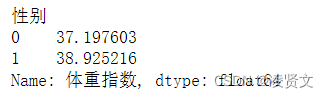## 7.2 计算每个患者与每个性别平均值的差异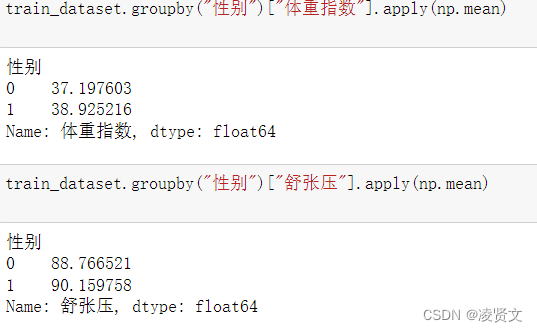"""人体的成人体重指数正常值是在18.5-24之间低于18.5是体重指数过轻在24-27之间是体重超重27以上考虑是肥胖高于32了就是非常的肥胖。"""def BMI(a):    if a<18.5:        return 0    elif 18.5<=a<=24:        return 1    elif 24<a<=27:        return 2    elif 27<a<=32:        return 3    else:        return 4    data['BMI']=data['体重指数'].apply(BMI)data['出生年份']=2022-data['出生年份']  #换成年龄#糖尿病家族史"""无记录叔叔或者姑姑有一方患有糖尿病/叔叔或姑姑有一方患有糖尿病父母有一方患有糖尿病"""def FHOD(a):    if a=='无记录':        return 0    elif a=='叔叔或者姑姑有一方患有糖尿病' or a=='叔叔或姑姑有一方患有糖尿病':        return 1    else:        return 2    data['糖尿病家族史']=data['糖尿病家族史'].apply(FHOD)data['舒张压']=data['舒张压'].fillna(-1)"""舒张压范围为60-90"""def DBP(a):    if a<60:        return 0    elif 60<=a<=90:        return 1    elif a>90:        return 2    else:        return adata['DBP']=data['舒张压'].apply(DBP)data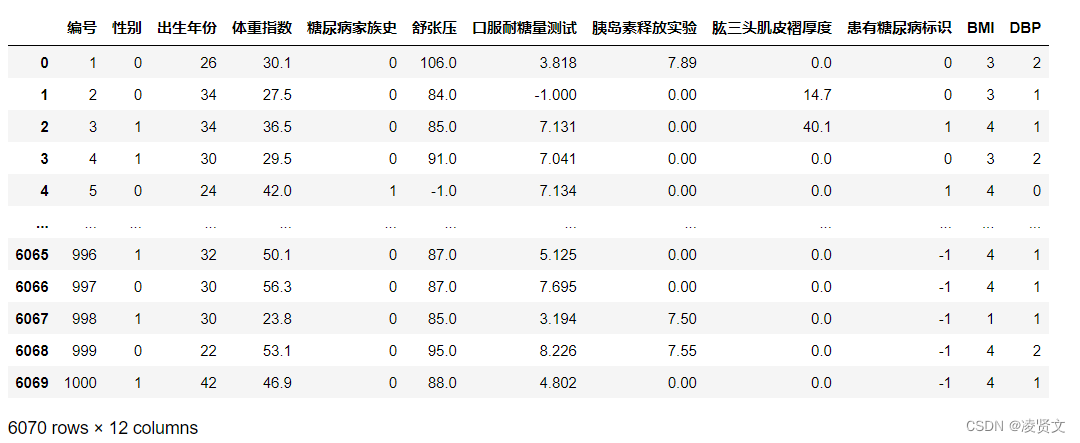# 八、高阶树模型

## 8.1 安装 lightgbm

import lightgbm as lgbimport pandas as pdimport numpy as npfrom sklearn.linear_model import LogisticRegressionfrom sklearn.tree import DecisionTreeClassifierfrom sklearn.preprocessing import MinMaxScalerfrom sklearn.pipeline import make_pipelinefrom sklearn.model_selection import train_test_splitfrom sklearn.metrics import accuracy_score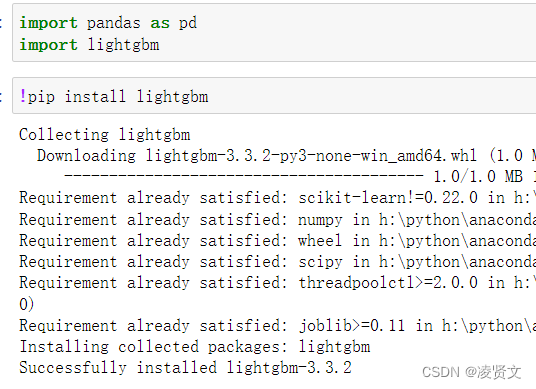## 8.2 将训练集 20%划分为验证集，使用 LightGBM 完成训练

train_data = pd.read_csv("比赛训练集.csv",encoding='gbk')test_data = pd.read_csv("比赛测试集.csv",encoding='gbk')train_data = pd.get_dummies(train_data)test_data = pd.get_dummies(test_data)# 划分数据集train_x,valid_x = train_test_split(train_data,test_size=0.2)clf_lgb = lgb.LGBMClassifier(    max_depth=3,     n_estimators=4000,     n_jobs=-1,     verbose=-1,    verbosity=-1,    learning_rate=0.1,)clf_lgb.fit(train_x.drop(["患有糖尿病标识"],axis=1),train_x["患有糖尿病标识"])predicts = clf_lgb.predict(valid_x.drop(["患有糖尿病标识"],axis=1))print(accuracy_score(valid_x["患有糖尿病标识"], predicts))[LightGBM] [Warning] verbosity is set=-1, verbose=-1 will be ignored. Current value: verbosity=-10.9546351084812623# 搜索参数kfold = StratifiedKFold(n_splits=5,shuffle=True,random_state=2022)classifier = lgb.LGBMClassifier()params = {    " max_depth":[4,5,6],    "n_estimators":[3000,4000,5000],    "learning_rate":[0.15,0.2,0.25]}clf  = GridSearchCV(estimator=classifier,param_grid=params,verbose=True,cv=kfold)clf.fit(train_x.drop(["患有糖尿病标识"],axis=1),train_x["患有糖尿病标识"])predicts1 = clf.best_estimator_.predict(valid_x.drop(["患有糖尿病标识"],axis=1))print(accuracy_score(valid_x["患有糖尿病标识"], predicts1))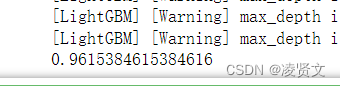# 九、多折训练与集成

import pandas as pdimport numpy as npimport seaborn as snsfrom sklearn.model_selection import KFoldimport lightgbm as lgb# 读取数据train_df = pd.read_csv('./糖尿病遗传风险预测挑战赛公开数据/比赛训练集.csv', encoding='gbk')test_df = pd.read_csv('./糖尿病遗传风险预测挑战赛公开数据/比赛测试集.csv', encoding='gbk')# 基础特征工程train_df['体重指数_round'] = train_df['体重指数'] // 10test_df['体重指数_round'] = train_df['体重指数'] // 10train_df['口服耐糖量测试'] = train_df['口服耐糖量测试'].replace(-1, np.nan)test_df['口服耐糖量测试'] = test_df['口服耐糖量测试'].replace(-1, np.nan)dict_糖尿病家族史 = {    '无记录': 0,    '叔叔或姑姑有一方患有糖尿病': 1,    '叔叔或者姑姑有一方患有糖尿病': 1,    '父母有一方患有糖尿病': 2}train_df['糖尿病家族史'] = train_df['糖尿病家族史'].map(dict_糖尿病家族史)test_df['糖尿病家族史'] = test_df['糖尿病家族史'].map(dict_糖尿病家族史)train_df['糖尿病家族史'] = train_df['糖尿病家族史'].astype('category')test_df['糖尿病家族史'] = train_df['糖尿病家族史'].astype('category')train_df['性别'] = train_df['性别'].astype('category')test_df['性别'] = train_df['性别'].astype('category')train_df['年龄'] = 2022 - train_df['出生年份']test_df['年龄'] = 2022 - test_df['出生年份']train_df['口服耐糖量测试_diff'] = train_df['口服耐糖量测试'] - train_df.groupby('糖尿病家族史').transform('mean')['口服耐糖量测试']test_df['口服耐糖量测试_diff'] = test_df['口服耐糖量测试'] - test_df.groupby('糖尿病家族史').transform('mean')['口服耐糖量测试']# 模型交叉验证def run_model_cv(model, kf, X_tr, y, X_te, cate_col=None):    train_pred = np.zeros( (len(X_tr), len(np.unique(y))) )    test_pred = np.zeros( (len(X_te), len(np.unique(y))) )    cv_clf = []    for tr_idx, val_idx in kf.split(X_tr, y):        x_tr = X_tr.iloc[tr_idx]; y_tr = y.iloc[tr_idx]        x_val = X_tr.iloc[val_idx]; y_val = y.iloc[val_idx]        call_back = [            lgb.early_stopping(50),        ]        eval_set = [(x_val, y_val)]        model.fit(x_tr, y_tr, eval_set=eval_set, callbacks=call_back, verbose=-1)        cv_clf.append(model)        train_pred[val_idx] = model.predict_proba(x_val)        test_pred += model.predict_proba(X_te)    test_pred /= kf.n_splits    return train_pred, test_pred, cv_clfclf = lgb.LGBMClassifier(    max_depth=3,     n_estimators=4000,     n_jobs=-1,     verbose=-1,    verbosity=-1,    learning_rate=0.1,)train_pred, test_pred, cv_clf = run_model_cv(    clf, KFold(n_splits=5),    train_df.drop(['编号', '患有糖尿病标识'], axis=1),    train_df['患有糖尿病标识'],    test_df.drop(['编号'], axis=1),)print((train_pred.argmax(1) == train_df['患有糖尿病标识']).mean())test_df['label'] = test_pred.argmax(1)test_df.rename({'编号': 'uuid'}, axis=1)[['uuid', 'label']].to_csv('submit.csv', index=None)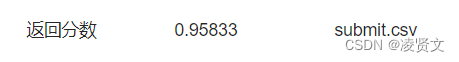## 评论